# SQL SUM() function

## SUM() function

The SQL AGGREGATE SUM() function returns the SUM of all selected column.

Syntax:

`SUM ([ALL | DISTINCT] expression )`

DBMS Support : SUM() function

 DBMS Command MySQL Supported PostgreSQL Supported SQL Server Supported Oracle Supported

DB2 and Oracle Syntax :

` SUM ([ALL | DISTINCT] expression ) OVER (window_clause)`

Parameters:

Name Description
ALL Applies to all values.
DISTINCT Return the SUM of unique values.
expression Expression made up of a single constant, variable, scalar function, or column name. The expression is an expression of the exact numeric or approximate numeric data type category, except for the bit data type. Aggregate functions and subqueries are not permitted.

Syntax diagram - SUM() function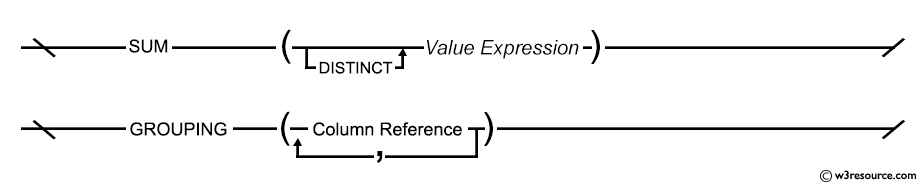## SQL SUM() on specific column example

To get the total SUM of 'advance_amount' of the 'orders' table, the following SQL statement can be used :

Sample table: orders

SQL Code:

``````SELECT SUM(advance_amount)
FROM orders;
``````

Relational Algebra Expression: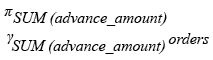Relational Algebra Tree: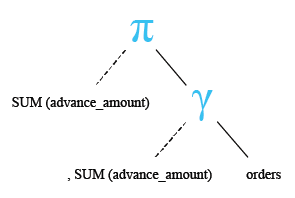Output:

```SUM(ADVANCE_AMOUNT)
-------------------
19450
```

Pictorial Presentation: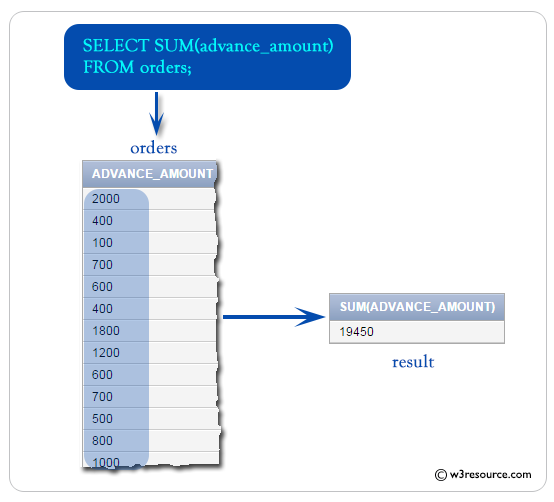## SQL SUM() using multiple columns example

To get the sum of 'opening_amt' and 'receive_amt' from the 'customer' table, the following SQL statement can be used:

Sample table: customer

SQL Code:

``````SELECT SUM (opening_amt + receive_amt)
FROM customer;
``````

Relational Algebra Expression: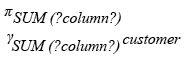Relational Algebra Tree: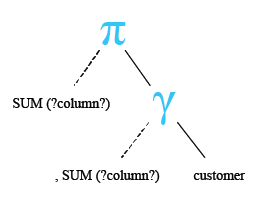Output:

```SUM(OPENING_AMT+RECEIVE_AMT)
----------------------------
353000
```

## SQL SUM() with where

In the following example, we have discussed usage of WHERE clause along with the SQL SUM() function to sum one or more columns against one or more conditions.

Example:

To get the total SUM of 'advance_amount' of the 'orders' table with the following condition -

1. 'agent_code' must be 'A003',

the following SQL statement can be used :

Sample table: orders

SQL Code:

``````SELECT SUM (advance_amount)
FROM orders
WHERE agent_code = 'A003';
``````

Relational Algebra Expression: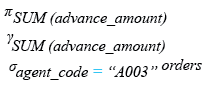Relational Algebra Tree: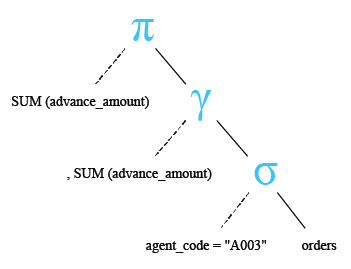Output:

```SUM(ADVANCE_AMOUNT)
-------------------
1000
```

## SQL SUM() with COUNT()

In the following example, we have discussed the usage of SQL SUM() and SQL COUNT() together in a SQL SELECT statement. Regarding this, it should be mentioned that the SQL SUM() and SQL COUNT() both returns a single row.

Example:

To get data of 'cust_country',SUM of 'opening_amt' for each 'cust_country' and number of 'cust_country' from the 'customer' table with the following condition -

1. data should be a group on 'cust_country',

the following SQL statement can be used :

Sample table: customer

SQL Code:

``````SELECT cust_country, SUM(opening_amt),
COUNT(cust_country)
FROM customer
GROUP BY cust_country;
``````

Relational Algebra Expression: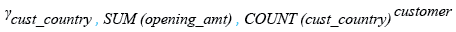Relational Algebra Tree: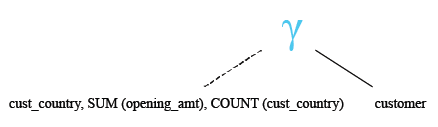Output:

```CUST_COUNTRY         SUM(OPENING_AMT) COUNT(CUST_COUNTRY)
-------------------- ---------------- -------------------
USA                             18000                   4
India                           73000                  10
Australia                       19000                   3
UK                              26000                   5
```

Note: Outputs of the said SQL statement shown here is taken by using Oracle Database 10g Express Edition

Here is a slide presentation of all aggregate functions.

Check out our 1000+ SQL Exercises with solution and explanation to improve your skills.

Previous: COUNT Having and Group by
Next: SUM using GROUP BY

﻿

## SQL: Tips of the Day

Difference between natural join and inner join

One significant difference between INNER JOIN and NATURAL JOIN is the number of columns returned-

Consider:

```TableA                           TableB
+------------+----------+        +--------------------+
|Column1     | Column2  |        |Column1  |  Column3 |
+-----------------------+        +--------------------+
| 1          |  2       |        | 1       |   3      |
+------------+----------+        +---------+----------+
```

The INNER JOIN of TableA and TableB on Column1 will return

```SELECT * FROM TableA AS a INNER JOIN TableB AS b USING (Column1);
SELECT * FROM TableA AS a INNER JOIN TableB AS b ON a.Column1 = b.Column1;
```
```+------------+-----------+---------------------+
| a.Column1  | a.Column2 | b.Column1| b.Column3|
+------------------------+---------------------+
| 1          |  2        | 1        |   3      |
+------------+-----------+----------+----------+
```

The NATURAL JOIN of TableA and TableB on Column1 will return:

```SELECT * FROM TableA NATURAL JOIN TableB
+------------+----------+----------+
|Column1     | Column2  | Column3  |
+-----------------------+----------+
| 1          |  2       |   3      |
+------------+----------+----------+
```

Ref: https://bit.ly/3AG5CId

We are closing our Disqus commenting system for some maintenanace issues. You may write to us at reach[at]yahoo[dot]com or visit us at Facebook